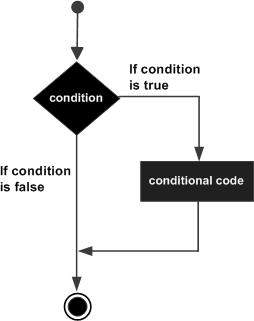# VB.Net - If...Then Statement

Advertisements

It is the simplest form of control statement, frequently used in decision making and changing the control flow of the program execution. Syntax for if-then statement is −

```If condition Then
[Statement(s)]
End If
```

Where, condition is a Boolean or relational condition and Statement(s) is a simple or compound statement. Example of an If-Then statement is −

```If (a <= 20) Then
c= c+1
End If
```

If the condition evaluates to true, then the block of code inside the If statement will be executed. If condition evaluates to false, then the first set of code after the end of the If statement (after the closing End If) will be executed.

## Flow Diagram## Example

```Module decisions
Sub Main()
'local variable definition
Dim a As Integer = 10

' check the boolean condition using if statement
If (a < 20) Then
' if condition is true then print the following
Console.WriteLine("a is less than 20")
End If
Console.WriteLine("value of a is : {0}", a)
Console.ReadLine()
End Sub
End Module
```

When the above code is compiled and executed, it produces the following result −

```a is less than 20
value of a is : 10
```
vb.net_decision_making.htm
Advertisements